# Machine Learning Summary (Algorithm)The left limb of the Lone Cypress is broken due to a big storm after the picture is taken.

In the last article, we look into the fundamental of ML and some complex ML algorithms, like EM algorithm, Hidden Markov Model, Bayes inference, etc… In this article, we dig into more traditional ML algorithms including regression, classification, kernels, cost functions, regularization, Gaussian Process, MLE, MAP, Bayesian linear regression, SVM, clustering and decision tree. Other topics including Laplace approximation, Lagrange multiplier, and Restricted Boltzmann Machine are also covered. We will go through some deep network like LSTM quickly. Again, to shorten the article, we will be brief on topics that people may be familiar with. In addition, we add some topics like normalization that may be more relevant to DL than ML. Again, the article is long. Please feel free to skip sections based on your level of interest.

### Regression

Linear regression

Model y=f(x) with a linear model parameter w.

Add a constant bias:

Standardization

In many ML algorithms or regression, we assume the input has gone through a pre-processing and is already standardized.

Residual Sum of Squares & Weighted Sum of Squares

To train a linear regression model, we define a cost function and minimize it, like RSS (residual sum of squares) which is called the least squares linear regression.

Change of basis with Polynomial regression

We can add new components to a new base in the polynomial form to expand the complexity of the model.

The added x₁² and x₂² components transform the non-linear decision boundary in the low dimension space into a linear boundary in the high-dimensional space. In theory, we can transform any boundary shape in low dimension into linear boundary in a higher dimension.

The generic form of the linear regression is

where g can be any function including non-linear, logarithmic, etc ... It is often mistaken that the linear regression models a linear function only. By expanding a basis, we can model complex and non-linear functions.

In ML, engineers apply domain knowledge to construct g that resembles the relationship between the input and the prediction. However, it is hard and we easily overdo it. To correct the problem, we can apply an L1-penalty. L1 cost favors sparsity in w (explained later). This allows the training to select fewer base vectors in making predictions. This mitigates the problem when too many input features are included and the model is overfitted.

Change of basis solution (Gram matrix)

The general solution for the linear regression after a change of basis is: (with proof later)

Parameter-based v.s. non-parameter-based

For the linear regression y = wᵀx, we assume a linear function for our model. This type of model is called the parameter-based model. The function is fixed but the function parameter will change with training data. For non-parameter-based model, we have no assumption on the function, we let the data to mold the function.

The kernel method is one of the non-parameter-based models.

Kernel

Previously. we generalize the linear regression as y = wᵀg(x). With the concept of the kernel method, we expand the linear regression further to explore the relation of x with other training data points x⁽ⁱ⁾ in making predictions. This allows us to mold a function based on the training data, rather than a pre-defined function.

Instead of making a prediction from wᵀx, we compute the similarity k between x and a training data point xᵢ. We multiply it with the corresponding model wᵢ. Then we sum up the results from all data points. Intuitively, we, sort of, compute a weighted average of the training data labels. For example, in predicting the salary from your years of education, we use higher weights for data points with similar years of education to you. Then we calculate a weighted average from the training data salaries.

The definition of a kernel is:

In general, a kernel map xᵢ and xⱼ into a high dimensional space and compute their inner product to explore similarity. But in practice, we can simplify the equation above to a form which simply measures the similarity of two data points, for example, using a Gaussian distribution.

However, the definition above is helpful in linking the Gaussian process with regression later. Next, we will detail this kernel method using RBF.

Radial basis functions (RBF — Gaussian Kernel)

RBF uses a Gaussian function for the kernel

A kernel function is symmetrical and the matrix is positive semi-definite. Sometimes, we take advantage of this property in making a proof. To train w, we use MSE loss function in our example.

To make a new prediction, we transform x into a matrix using the kernel function.

RBF computes how far the input from each training data point and multiply it with the corresponding wᵢ. i.e. we are computing a weighted average of the output based on similarity.

### Classification

Classification with regression

We can use Bayes’ Theorem to check whether the input x belongs to class y=0 or y=1. For example, if it should be label as y = 1, we want p(y=1|x) > p(y=0|x). Alternatively, we compute a linear regression xᵀw + b and use the sign to determine whether y belongs to 0 or 1. As shown below, if p(x|y) is Gaussian distributed, these two approaches are actually the same.

However, xᵀw is unbounded while we want our predictions to be -1 or 1. The choice of the cost function has to be careful here. As shown below, the least square cost function with xᵀw as input can add penalty into the cost function even it correctly predicts the class for x with no ambiguity.

Logistic regression will be introduced next to solve it or we can switch to cost functions like the hinge loss or the logistic loss.

Logistic regression

We can apply the result of the xᵀw to a logistic function (also called the sigmoid function) before making a decision. This squeezes the unbounded xᵀw between 0 and 1.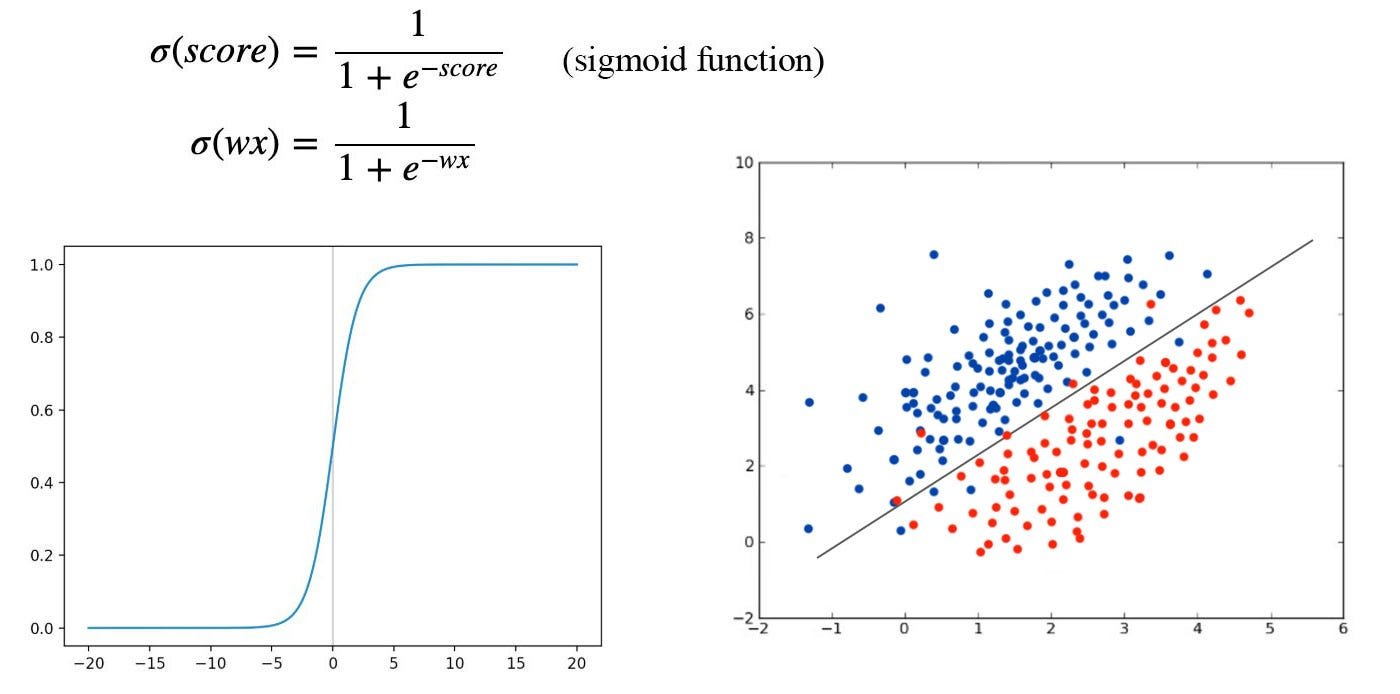Classify the data points according to σ(wx) ≥ 0 or not.

As shown below, the logistic function is related to the log odds (logits). Actually, we can derive the logistic function from logits. In this example, we use xᵀw to compute the score for the logistic function but other scoring methods including a deep network can be used.

Bayes classifier

Bayes classifier applies Bayes’ Theorem to classify x as below:

### Cost Functions & Regularization

Mean Square Error (MSE)

MSE is popular because it is easy to compute with a smooth differential. But it is vulnerable to outliners. We penalize large error un-proportionally high. If we have bad noisy data, we pay too much attention to fit these data into the model which we should ignore at the first place. MSE cost function for linear regression is

The corresponding optimized analytical solution (Normal equations) is

The proof can be found here. We will quote or use this equation very often.

MSE with an L2-regularization is called ridge regression. The corresponding optimal solution is

The following is the Gradient descent using the least mean square error (LMS).

L0, L1, Huber Loss or Regularization

Huber loss combines an L2 loss in the low error range with an L1 loss for the high error range. This combines the derivative smoothness of L2 and the smaller vulnerability to the outlier in L1.

L1 & L2 Regularization comparison

L2 has a smoother gradient and therefore the training can be more stable. But L1 is also popular because it promotes sparseness. As shown below, the optimal point after adding an L1 regularization favors parameters to be 0. It encourages spareness in avoiding overfitting.

If an L1-regularization is used with the least square error, the linear regression is called Lasso regression. While the choice depends on the specific data and problem domain, L2-regularization seems more popular but feel free to experiment both.

Convexity

Let’s explore the corresponding optimal solution for different objective functions with different p-norm.

• If we don’t have any regularization (λ=0), the optimization can be solved analytically if XᵀX is invertible.
• If p=2, an analytical solution is guaranteed.
• For p≥1 but not equal to 2, we can use an iterative method to find the global optimal.
• When p<1, the objective function will not be convex. In contrast to other choices, global optimal is not guaranteed. We can only find a local optimal with numerical methods.

Cross-entropy

In ML, we want our predictions to match the ground truth which P is the ground truth and Q is the model prediction. For classification, P(xᵢ)=1 for the ground truth label i and P(xⱼ)=0, otherwise.

Softmax function

For multiple-class classification, we can use cross-entropy with Q(x) computed form the softmax function. In linear regression, the input score for the softmax function equals xᵀw for the corresponding class.

KL Divergence

KL-divergence measures the difference between two data distributions.

Note: KL-Divergence is not symmetrical and it is always greater or equal to 0.

Jensen-Shannon Divergence

Jensen-Shannon Divergence is symmetrical.

Logistic loss

It measures the loss based on the logistic function. So we can apply a logistic function to the output and use the cross-entropy loss or we can use the logistic loss in the objective function instead.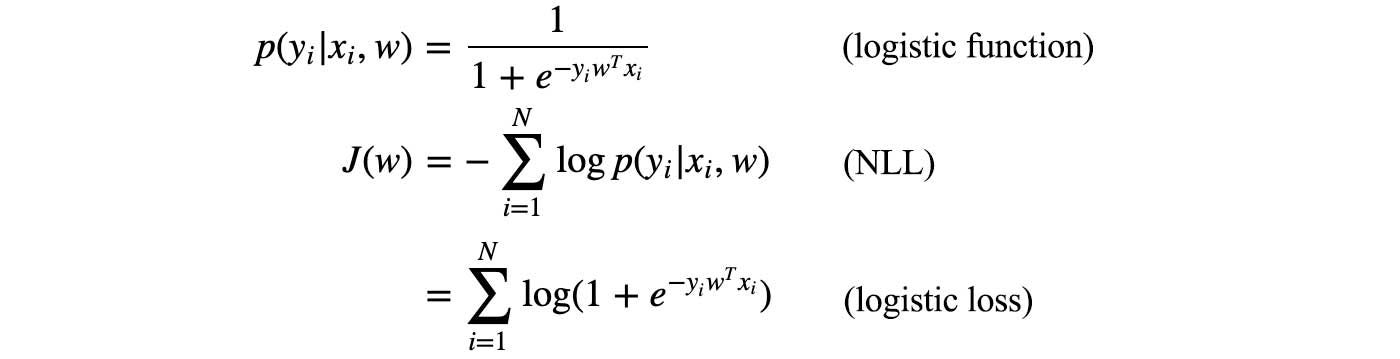Proof

Hinge loss

Hinge loss penalizes classification mistakes only if the prediction is wrong or too close to the decision boundary. Hinge loss is used in SVM and the details will be discussed later.

Recap

### Model Optimization

Maximum Likelihood Estimation (MLE)

Find the model parameters that maximize the likelihood of the observed data xᵢ.

Negative log likelihood (NLL)

MLE has a long chain of multiplication which is vulnerable to diminishing or exploding problems. To solve the problem, we take a logarithm function on the objective function. Since “log” is a monotonic function, maximizing MLE is the same as minimizing the negative log likelihood (NLL).

In optimizing an objective, we can add (subtract) or multiply (divide) values as long as it is invariant w.r.t. the model parameters. We can also add a monotonic function. The optimal solution remains the same.

As shown below, NLL is also the same as optimizing the cross-entropy.

If p(y|θ) can be modeled by a zero-centered independent Gaussian Distribution, we can prove maximizing MLE is equivalent to minimizing MSE.

Intuitively, the square part in the exponential function of the Gaussian distribution contributes to the reason MLE is the same as optimizing the least square.

MLE with linear regression

Let’s say w is the ground truth weight for the linear regression model yᵢ = Xᵢᵀwᵢ + εᵢ where εᵢ is the noise with variance σᵢ². The observed y can be modeled as

If we sample data and use MLE to train w, how good will w be? Below is the expected value and the covariance for our estimation on w using MLE. (Feel free to spend some time to study the proof if you are interested in how expectation and variance are calculated in an estimator.)

As indicated, the estimated w using MLE objective is unbiased. However, if σ²(XᵀX)⁻¹ is large, the variance is high. You get very different results using different training dataset. In short, the estimated w values are sensitive to the noise in the measured data. This is a good example that even your estimation is unbiased, the model is no good if it has a high variance. Let’s get into the reason a little bit more.

Every matrix X can be decomposed using SVD as

(XᵀX)⁻¹ is proportional to the inverse of S² where S is a diagonal matrix with diagonal elements containing the singular values of X. So if some of the singular values are small, the variance σ²(XᵀX)⁻¹ is high. Therefore, by evaluating the singular value of X, we understand the variance of a model.

Small singular values happen when the columns in X are highly related.

Intuitively, when information is highly correlated, the estimation is vulnerable to variance and the model is easier to be overfitted.

To address this issue, we can add L2 regularization (Ridge regression) to constrain the model parameters. This regularization stabilizes the inverse numerically.

The trained w using ridge regression isSource

When Sᵢᵢ is very small, λ comes into the rescue and the individual element in S⁻¹ will have a certain upper bound. Otherwise, it will be unlimited large and magnify slight variation of the data and cause high variance in w.

The equation below compares the difference of w trained with ridge regression and least square alone.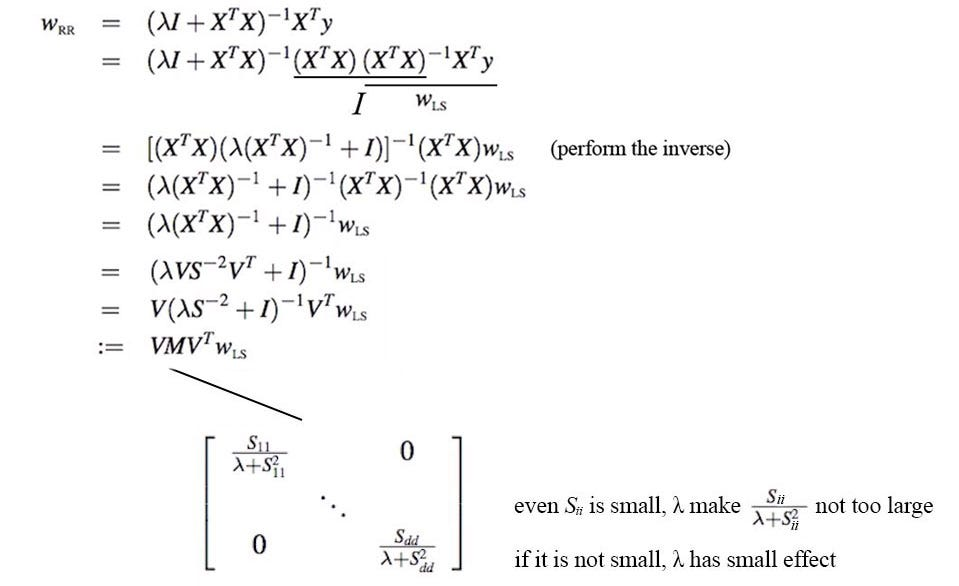Modified from source

Again λ limits how far we are from the least square solution. The expected value and the variance for the ridge regression is

MLE is unbiased but it may have high variance. Ridge regression is biased but has lower variance. Because we can always tune λ, we can tailor a solution sensitive to the data distribution.

Optimization in alternative steps

In many situations, we need to break down machine learning problems into iterations of two alternative steps which one optimizes a sub-set of parameters and the other step optimizes the rest. The EM-algorithm discussed in the previous article is one of the examples. In these problems, we cannot optimize both sets of parameters simultaneously (or it is too hard or very unstable). These two sets of parameters often have inter-dependency between them and we cannot compute their derivative simultaneously. Because of such inter-dependency, we optimize one set at a time while fixing the other.Source

In each step, the cost drops and the solution will converge to a local or global optimal. However, for a non-convex objective function, we will unlikely reach the global optimal but in practice, many problems still produce good results.

This algorithm takes advantage of the monotonic decrease in cost. Within certain precision, the space for θ₁ and θ₂ are in limited size. We cannot keep decreasing cost forever and therefore, the solution will converge. Later, we will demonstrate this type of algorithms with specific examples.

Maximum A Posteriori (MAP)

Previously, we take the MSE for granted and use it as the cost function to train a model. MAP can be used to optimize a model or to justify such a decision. Instead of optimizing θ to maximize p(y|θ, x) in MLE, we optimize θ to maximize p(θ | y, x).

To calculate p(θ | y, x), we apply Bayes’ Theorem.

If we assume the model parameters θ are zero centered, and p(θ) and p(y|θ) are both Gaussian distributed, MAP justifies the use of LS (Least square) and L2 regularization as an objective function.

In short, we apply a prior belief that θ is Gaussian distributed. Combined with a likelihood of y (observation) that is modeled by another Gaussian model, we reach the same objective as the Ridge regression.

In summary, two different approaches in training a model w are discussed in the last few sections. One approach is based on the log-likelihood (or MLE) and the second one is based on MAP (with Bayes’ Theorem) in maximizing the posterior of w.

Newton’s Method for optimization

We can apply Newton’s Method iteratively to locate the lowest cost.

It is an alternative to gradient descent which only uses the first-order derivative. It is more accurate by using the curvature of f (f’’). However, it is computationally intense. Some kind of approximation is needed to make it practical for large scale problem.

Taylor series

Using Taylor series, we can expand and approximate a function f. In the example below, we expand f to the second order.

By differentiating the equation above w.r.t. ϵ, the optimal step ϵ* in minimizing f equals

Nash Equilibrium

In the game theory, the Nash Equilibrium is reached when no player will change its strategy after considering all possible strategy of opponents under a non-cooperative environment. Both opponents treat each other hostile or there is no mean for them to communicate their actions. Consider the possible jail time below on how Peter and Mary may confess to a crime they commit. It makes sense for both to keep quiet since it is the lowest possible jail time. But to reach a Nash Equilibrium under a non-cooperative environment, both will confess and get 6-month jail time instead of 1-month jail if both keep quiet.

Lagrange multiplier (Constrained Optimization)

In some ML or DL methods, we want to optimize a function subject to constraints.

Consider the cost function f=x+y and the constraint h (in red) below. (Example is adapted from here.)

To enforce constraint, we move along the orthogonal of the gradient of h. To reduce cost, we follow the negative gradient of f.

The equilibrium (the optimal solution) happens when both gradients are along the same line, i.e.

It does not need to be in the same direction because h(x) = 0 implies -h(x) = 0. So direction does not matter for equality constraint.

Next, we define the Lagrangian as

If we take the derivative of the Lagrangian w.r.t. x and μ respectively and set them to zero, we enforce the optimal point described before as well as the constraint.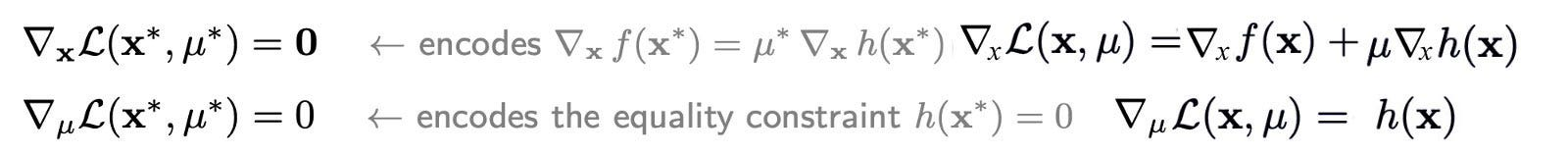Modified from source (Note, for the first equation, we absorb the negative sign into μ)

The first equation minimizes 𝓛 w.r.t. x to find the minimum cost for certain μ. The second equation maximizes 𝓛 w.r.t. μ to enforce the constraint (any constraint violation receives positive costs). Our objective here is to minimize the Lagrangian 𝓛 w.r.t x while maximizing 𝓛 over μ. Here is an example in solving a constrained optimization problem using Lagrangian.

We can have multiple constraints by having multiple Lagrangian multipliers μᵢ. To find the solution, we differentiate 𝓛 w.r.t. different μᵢ.

We can have inequality constraints in the Lagrangian also. Now the constraint requires the optimal point to be in the shaded area.

The Lagrangian is

The solution is similar to the equality constraint. To satisfy the inequality condition, we need to impose additional conditions below.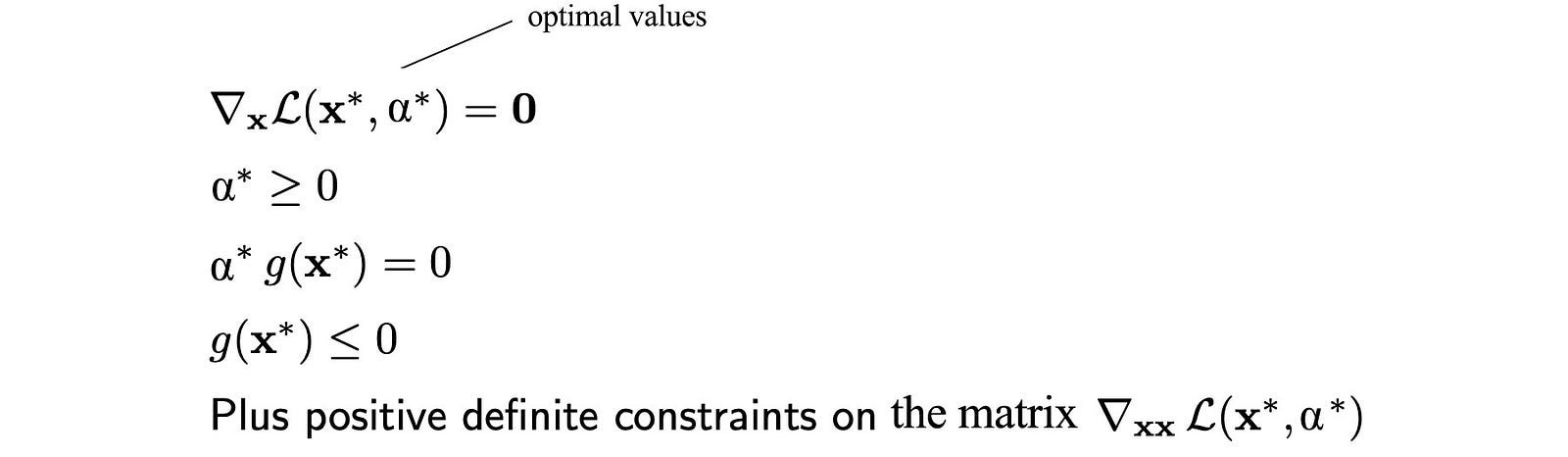Modified from source

### Generative learning v.s. discriminative learning

In DL, we design a deep network to predict a label y from data x. Generative learning instead builds a model for x given y. As shown by a Naive Bayes’ classifier, some problems are easier to model p(x|y) than p(y, x).

In generative learning, we can apply Bayes’ Theorem to make a prediction of p(y|x) from a model of p(x|y).

In general, generative learning can be expanded as the study of p(x). In some perspective, it is a study of generating data. In GAN, we create a generator to create x from sampling a noise z. It models p(x|z). In a Gaussian mixture model, we model p(x) using a mixture of Gaussian distribution.

### Gaussian

Gaussian discriminant analysis (GDA)

Say, we want to classify whether an image is an apple or an orange. We can use Bayes’ theorem with both p(x|y=apple) and p(x|y=orange) modeled by multivariate Gaussian distributions. (Credit: the equations in this example is adapted from here.)

With a 100 × 100 image, we get 100 × 100 × 3 features (RGB for each pixel). Using the images from the training dataset, we compute the mean of each feature from the same spatial pixelᵢⱼ in each image. μ₀ below is a 30,000-Dimensional vector contains the means of these features given the object is an apple. We repeat the same process for the orange in calculating μ₁.

Even we have 2 different vectors for the feature means of the apple and the orange, the model example here uses one covariance Σ created from the combined data. Therefore, the shapes for both distributions are the same.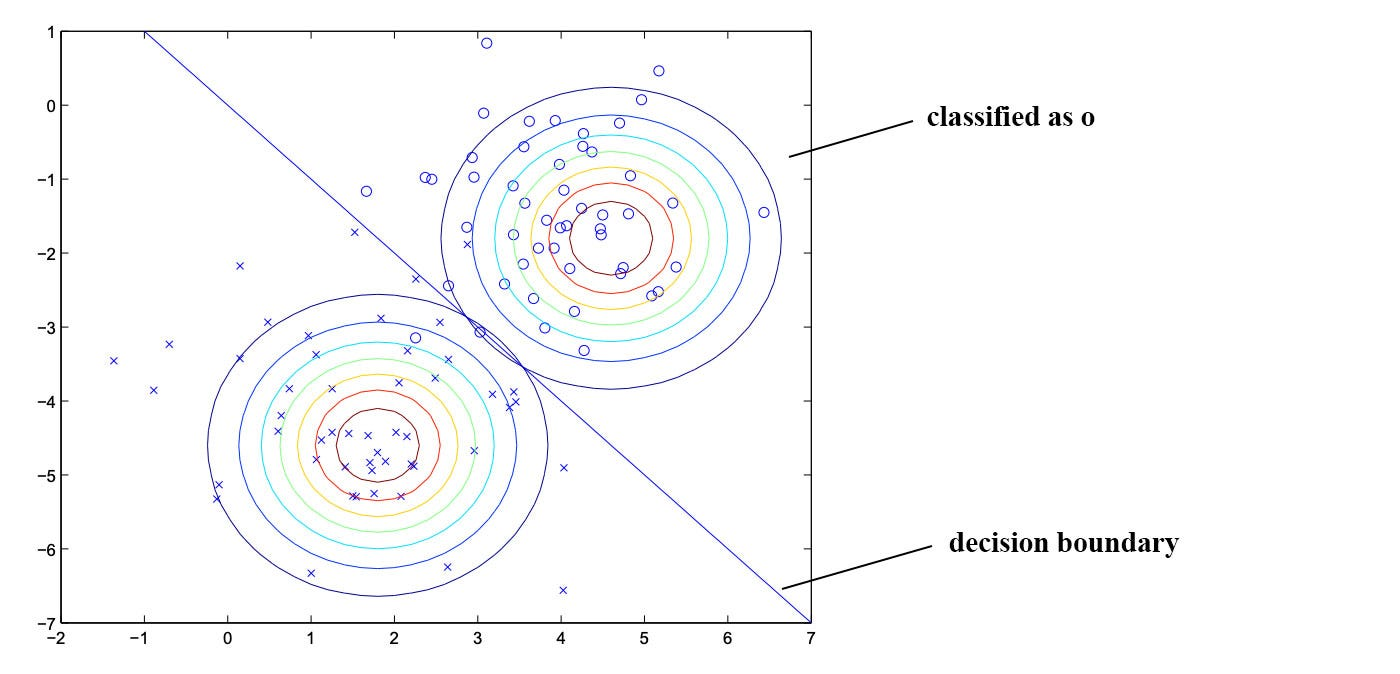Modified from source

We can draw a decision boundary between the two modes in dividing the classes.

However, if different variances (Σ) are used, the decision boundary will be non-linear. In fact, subject to the shapes of Σ, it can get very complex.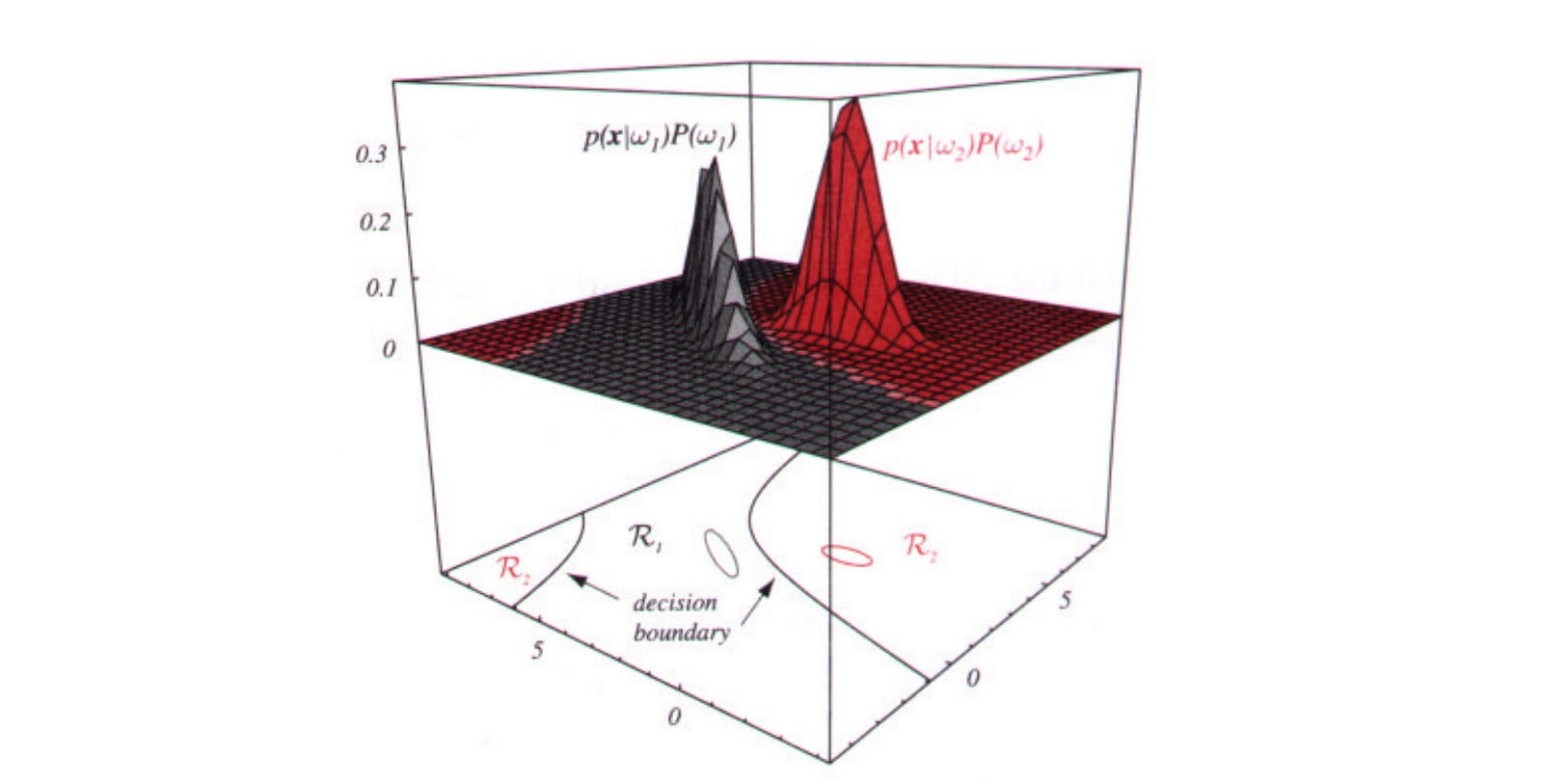Source

Bayesian linear regression

As we mentioned before, Bayes’ Theorem may look deceptively simple. But if we can model the prior and the likelihood with a Gaussian Distribution, the posterior will be Gaussian distributed and can be calculated analytically pretty easily.

Let’s combine linear regression and Bayes’ Theorem to make predictions. Our objective is to find model parameter θ using MAP.

So given the Gaussian model for the likelihood and prior below, we can formulate the posterior as:

The formula above is actually a Gaussian distribution even it is not obvious. So, let assume the posterior is Gaussian distributed with μ and Σ, and we will prove that we can solve both parameters.

We can expand a Gaussian definition into

By comparing it with our equation before, we find μ and Σ to be:

Predictive distribution

To make predictions, we can marginalize over the posterior distribution, a.k.a. ∫p(y|w)p(w)dw).

Again, we can solve that analytically without the integral.

With Bayes inference (the diagram on the right below), the uncertainty increases as the predictions have input far away from the circled training data.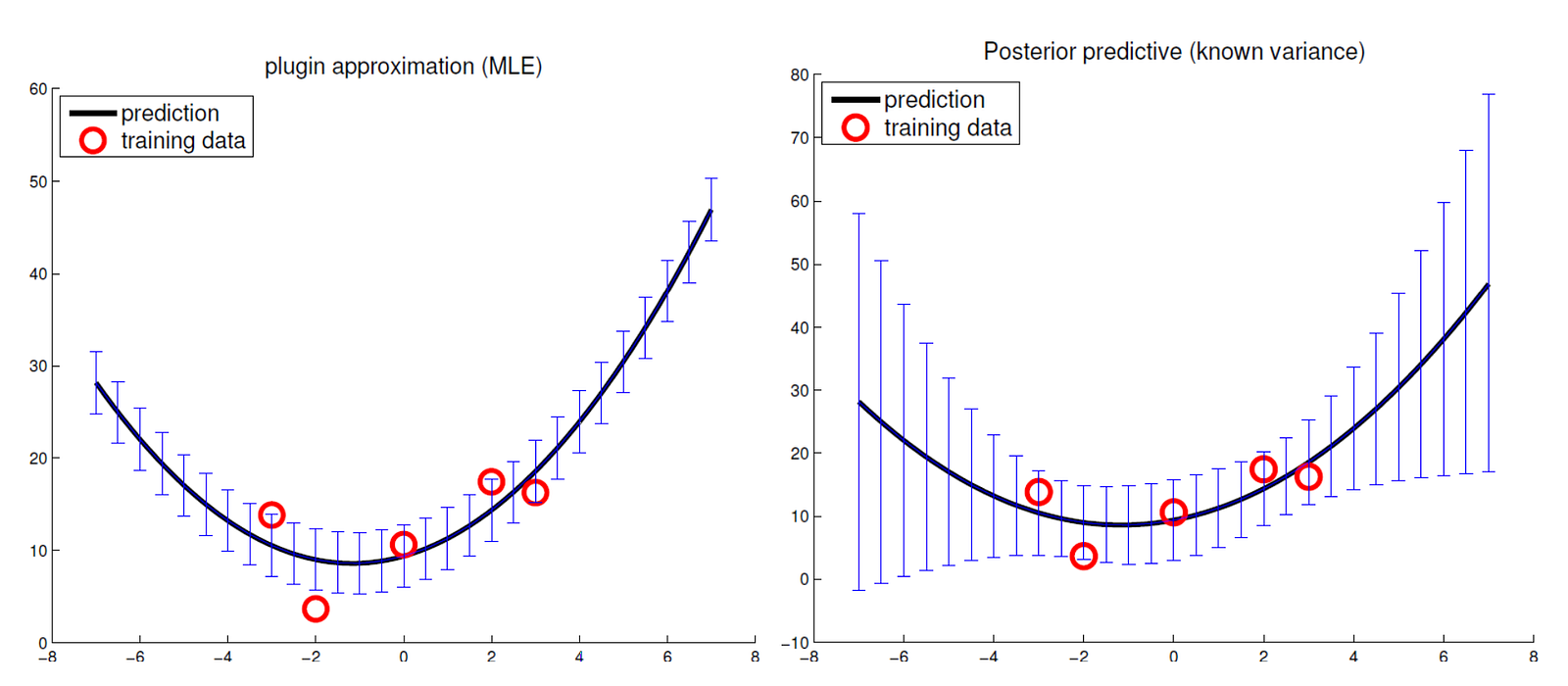Source

For point estimation algorithm, we don’t know how certain about our predictions. Bayes inference gives us a bonus on how confident we can be by continue tracking parameters and information with probabilities and distributions.

Active Learning

Measurements can be expensive and hard to perform. Active learning uses the current training dataset in determining what should be measured next (x₀ y₀). We want to pick addition training points x₀ with the best return in building up a model.

Let’s go through it step-by-step.

1. Calculate the predictive distribution above for the un-observed x₀ using the existing model.
2. Pick an x₀ which σ₀² is the largest and measure the corresponding y₀.
3. Update the posterior p(w|y, X) with the training dataset including the old and the new measurement (x₀, y₀) using the Bayesian linear regression.
4. Iterate the steps above again to build up a model.

The entropy of a Gaussian distribution is

Our selection of x₀ will drop the entropy of the posterior the most from its prior.

(Credit: Equations are adapted from Active learning in here.)

Laplace Approximation

As shown before, we can use Gaussian distribution to model the prior and the likelihood to calculate the posterior distribution. But it is not necessarily true that both are Gaussian. But even those are false, we can still assume the posterior is Gaussian distributed and calculate the distribution using the Laplace approximation with the posterior as

Let’s compute the posterior with MAP and define f as

Take a short note that optimizing MAP is the same as optimizing f since after integrating over w in the denominator, it is a constant w.r.t. w, and the exponential function in the numerator is a monotonic function.

We can approximate f with the Taylor expansion up to the second order.

The secret of the Laplace approximation is the choice of z. Here, we can maximize the log posterior (MAP solution) say using the gradient descent method. We will use this optimal weight w* as z. As mentioned before, optimizing MAP is the same as optimizing f. Therefore, f (z) should equal to 0. The posterior distribution can, therefore, be further simplified with

i.e.

We can recognize that the R.H.S. fits the definition of a multivariate Gaussian distribution where

And if we use logistic regression σ(yᵢxᵢᵀw) to compute p, the negative inverse of Σ is:

Put it together

Here is the final algorithm where λ is the regularization factor.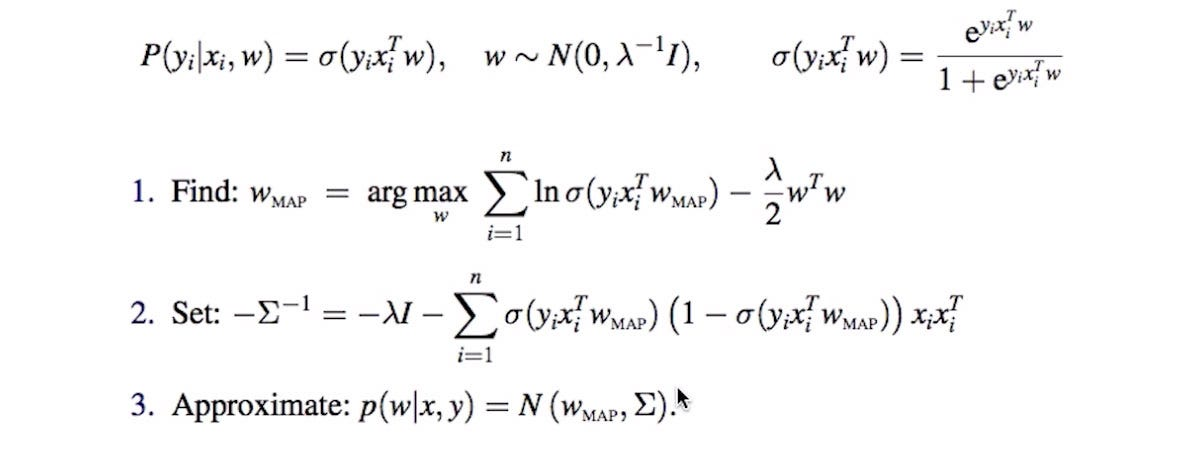Source

In step (1), we can use a gradient method to optimize a regularized log posterior using logistic regression. Then, we compute the covariance of the posterior in step (2).

### Gaussian Process

Conditional distribution in Multivariate Gaussian

Let x to be an n-dimensional vector which can be modeled with n means and a covariance matrix using Gaussian Distribution below.

We break x into 2 subvectors x₁ and x₂ and break μ and Σ into the corresponding components accordingly.

Now, we want to express the conditional probability of x₁ given x₂ in terms of the sub-components above.

This gives us a foundation of making predictions given some training data. In specific, given x₂ and its labels, we can make predictions for f(x₁). The general idea is to model the data distribution of f(x) and use it to make predictions. Let’s give an example.

Intuition

Consider we have the weight and height of two persons (person₁ (150, 66) & and person₂ (200, 72)). Let’s build a Gaussian model with this information. First, we use the height information to build a covariance matrix to quantify the similarity of person₁ and person₂. Then the weight of both person can be modeled with a Gaussian distribution with mean 175 (the mean of both people).

If we draw samples from this Gaussian Distribution, the expected value for these two persons should be 150 and 200 respectively. Next, we will build a function with height as an input in predicting weight.

First, we expand the Gaussian distribution above to make 2 weight predictions (f₁ and f₂) for person₃ and person₄. With the height information for all four persons, we compute a new covariance matrix. This matrix quantifies the correlation between these four persons. We can sample from the new distribution to make predictions on the unknown weight for the person₃ and person₄.

Why should we stop with two predictions above if we can make predictions for a large range of height values hᵢ?

When we sample from Ɲ, we got a function that maps height (from h₁ to hn) to weight, like the blue line above even though it looks odd. We can repeat the sampling in producing many functions. Therefore, the Gaussian process is considered as generating a distribution over functions.

For example, the left diagram below demonstrates 5 such sampled functions (5 curves in different colors) for another problem.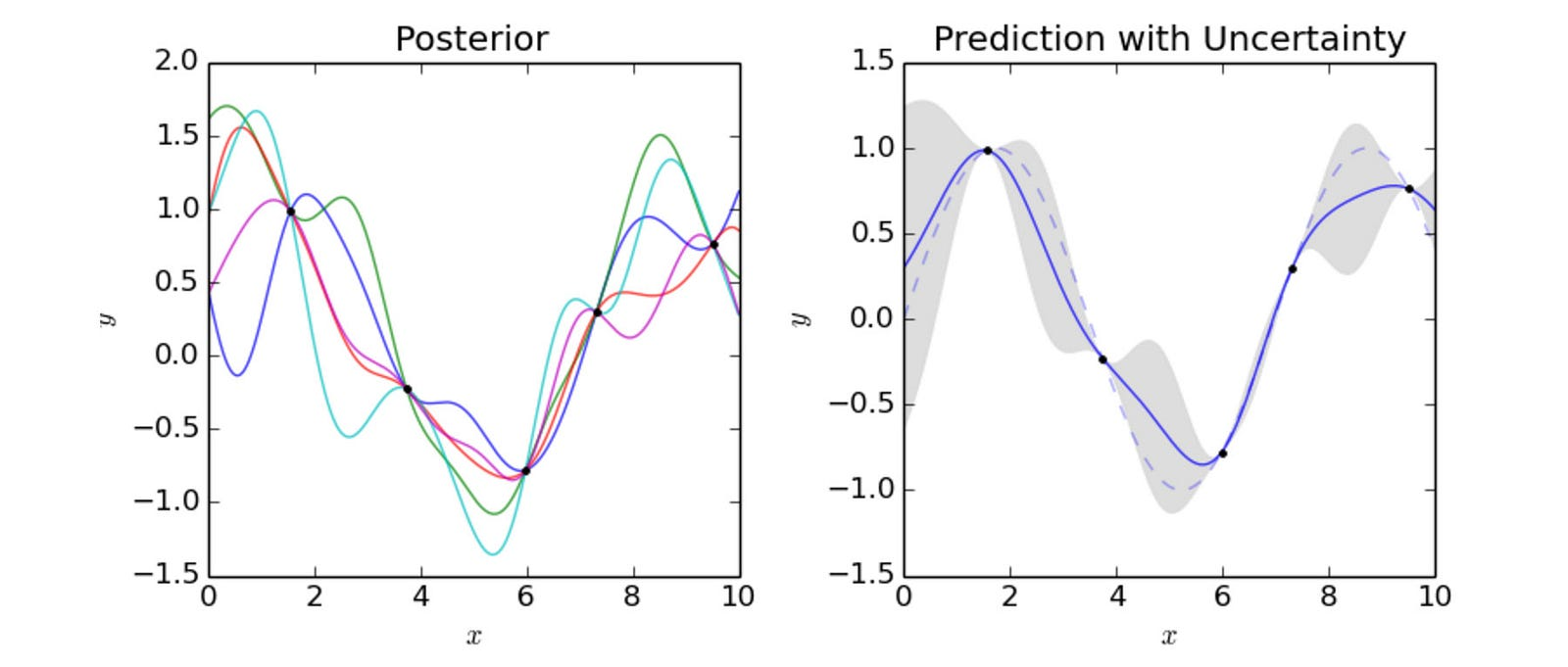Source

However, we are not interested in a particular function. The diagram on the right above shows the expected prediction and the shaded area is within one standard deviation. We are not making a point estimation any more. For a particular x, we calculate the expected prediction (a dot on the blue line on the right) and its variance (our likely range in our prediction). As shown above, as we move away from data points with known labels, the uncertainty of our prediction increases.

Gaussian process formula

Let’s work through the equations. Consider

f is the distribution generated from the training data. f* is what we want to predict. Given the training data, we want to compute the data distribution for

where f* will be modeled as

With the conditional distribution computed before, we compute the new mean and the covariance as

with

μ and μ* are the means for f and f* respectively (μ = 175 in our example). If our training data set is large enough and we are predicting data with a similar range as f, we can assume μ = μ*. Furthermore, if f is zero-centered, we can simplify the equation further to

However, finding the inverse of K can be numerically unstable. Therefore, we decompose K with Cholesky decomposition first and solve the problem using linear algebra (details). A sample program in demonstrating the calculation can be found here.

In summary, we use the height information to build a covariance matrix in quantifying correlations between people. We break up this matrix into sub-components and use that to recreate the weight distribution given the known labels in the training dataset.

Bayesian Linear Regression & Gaussian Process

Bayesian linear regression models its model parameter w with a Gaussian distribution and its observation y with another Gaussian with Xw as means and added noise with variance σ². For the Gaussian process, the variances come from the noise and the covariance matrix K. Actually, both methods are very similar.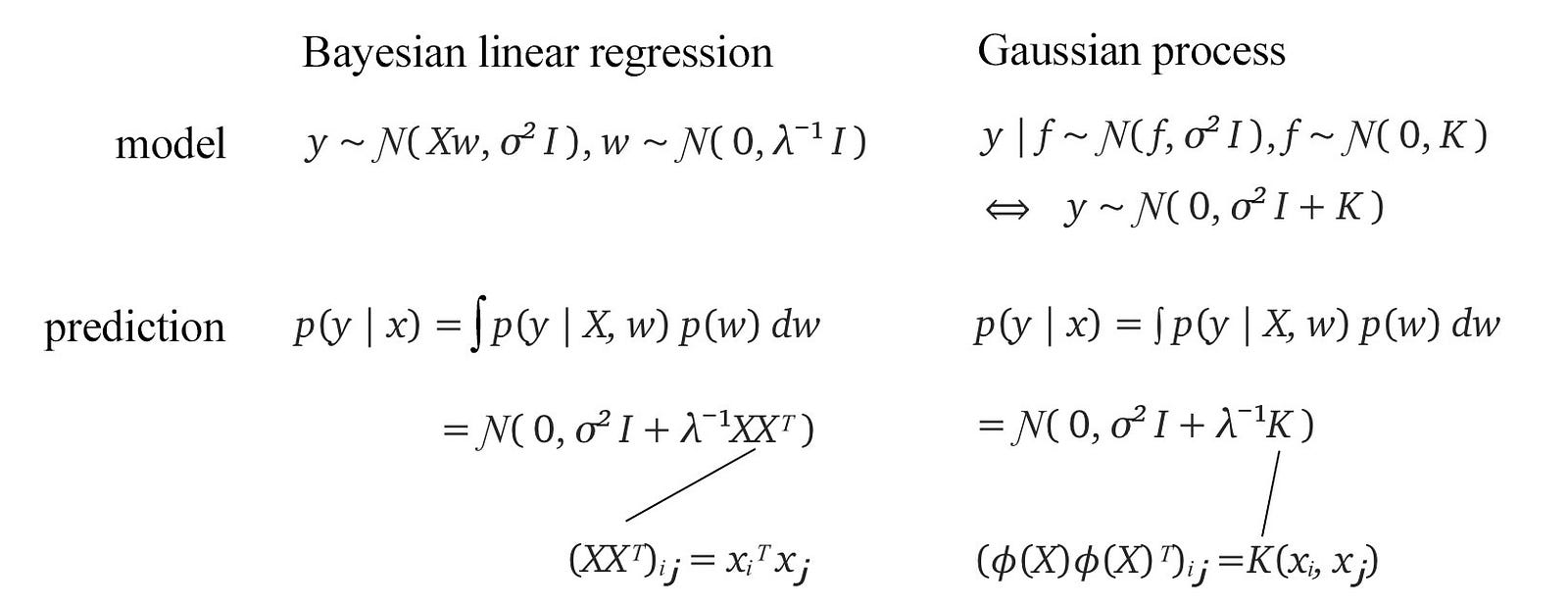Source (Gaussian Process) of the equations

In our example here, we further simplify the prediction y to be zero-centered. As we compare both predictions, the difference is one using the covariance matrix and one with the kernel. In short, the Gaussian process can be conceptualized as kernelized Bayesian linear regression. Instead of computing xᵢ xⱼᵀ, we replace it with a kernel.

Here is the mean and the variance for a new prediction y₀ using both methods.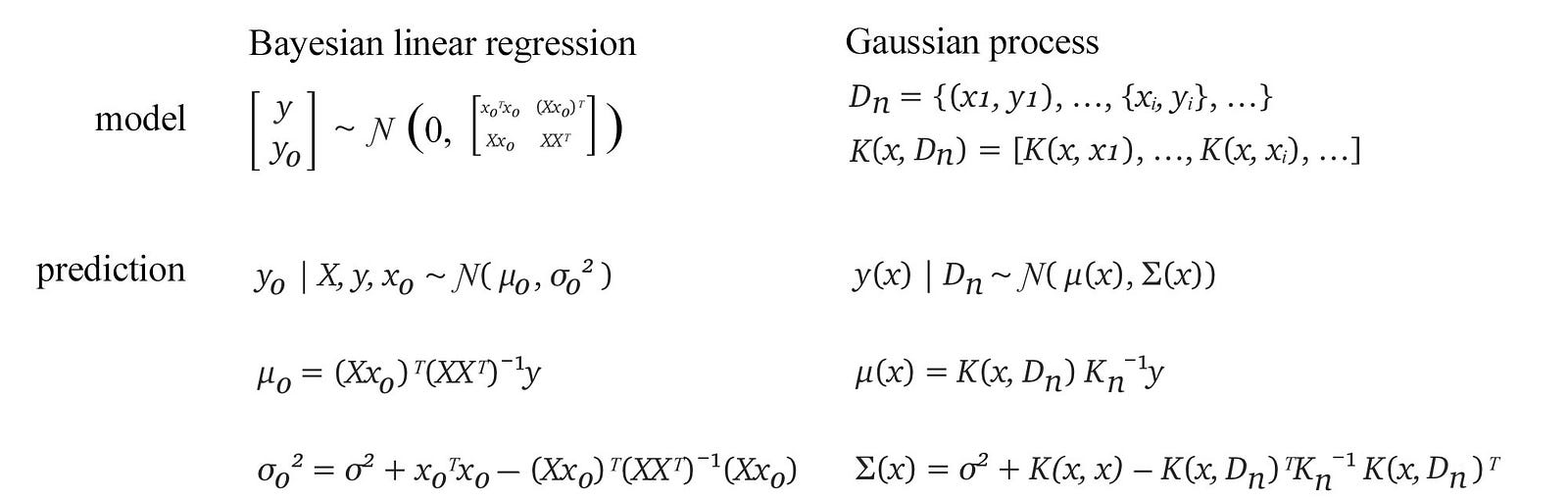Source (Gaussian Process) of the equations

### Support vector machine (SVM — classification)

In Gaussian-based methods, we model the data distribution for each class (i.e. p(x|y)). This creates a decision boundary in classifying data. SVM classifier adopts a different approach without discovering the distributions. It separates data points into one of the two corresponding classes through linear regression and hinge loss. SVM will maintain the largest possible margin in separating both classes.

If wᵀxⁱ < 0, x belongs to the green. Otherwise, it belongs to the red. When hinge loss is applied, we care about points that are close to the opposite class only (support vectors). Points that are away from the margin boundary incur zero cost as we already classify them correctly and they are not close to the decision boundary. We do not need to do better for those points and therefore they should have no impact on the model to be built. We only penalize a prediction that is wrong or close to being on the wrong side. The first term below is the hinge loss.

It adds a loss for every point x with yⁱwᵀxⁱ < 1 — points that violate the margin constraint.

The following is the mathematical objective for SVM. It maximizes xw ≥ 1 (for y=1) with the smallest w possible.

With this objective, SVM maximizes the margin of its decision boundary. Also, the trained w will be perpendicular to this decision boundary.

At a high level, SVM wants xᵀw ≥ 1 (for y=1) with the smallest possible w. The first condition is equivalent to pw≥ 1 which p is the projection of x on w.

To minimize ‖w‖, we want to increase p for the first condition. The projection of the blue dot is shown as the green line along w below. In the left diagram below, it has a small margin boundary that cut through two supporting vectors. To increase p, we can rotate w clockwise.

Effectively, as shown above, it also increases the margin. While our SVM objective tries to increase p, it has the same effect of increasing the margin.

If u and v are two support vectors having the shortest possible distance between two class boundary, the optimal solution for SVM w* will lay on uv and this solution has the largest margin. We had gone through the reason why SVM maximizes the margin graphically. If you feel it is not foolproof, it is normal because the thorough mathematical proof is more complicated but quite interesting in applying the concept of convexity and Lagrangian multiplier.

However, to avoid overfitting, we tune the regularization factor λ to mitigate noise in the training data.

SVM with kernels

We can further modify SVM to use a kernel output as the input.

The mathematical model for the SVM with constraint violation and kernel can be found here.

### Normalization

In general, to train a model, we standardize the input features first to make the model easier to train.

Next, we will study more normalization techniques that may be more relevant to DL than ML.

Batch normalization

Why do we perform normalization on the input only if we can normalize input in every layer in a deep network?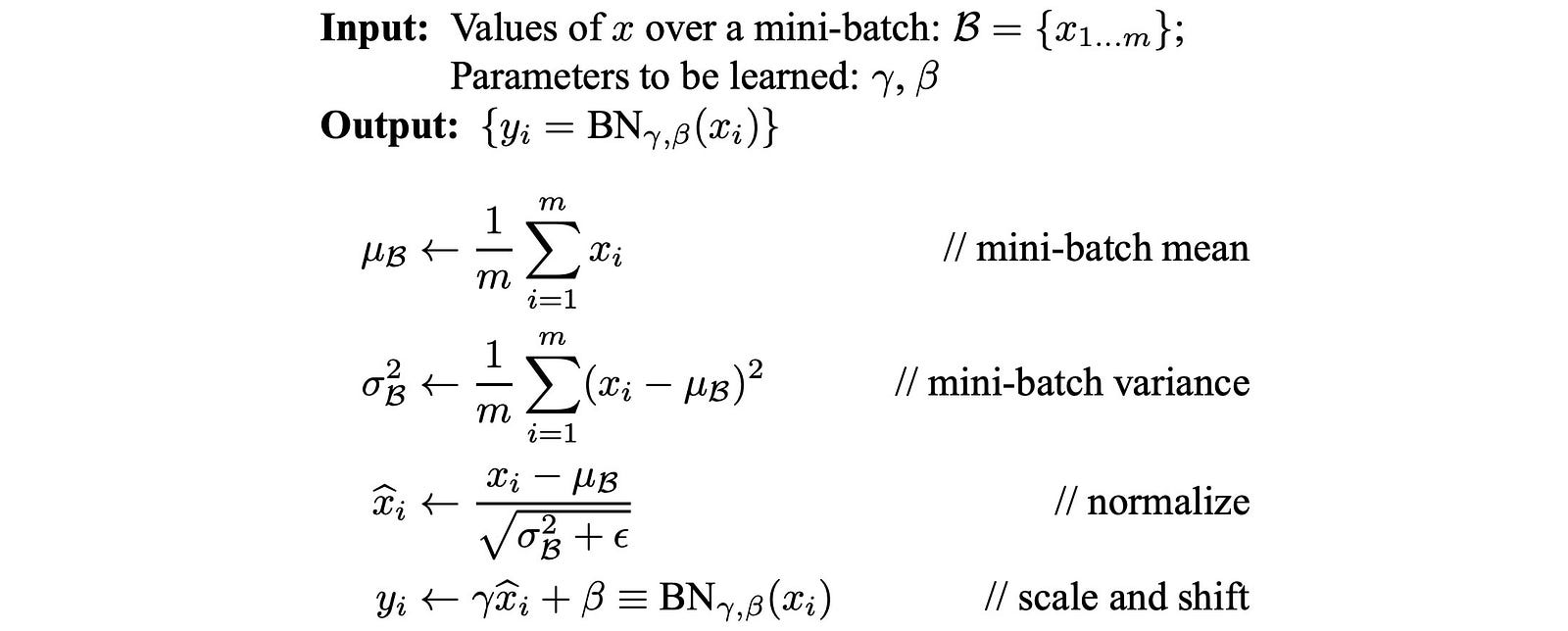Source

The first two steps simply calculate the mean and variance from the mini-batch. Note: during inference, we continue to use the mean and variance of the training dataset to maintain the statistic used in training the model. Batch normalization introduces trainable γ and β. This normalization can be undone if γ=σ and β=μ above. However, we initialize γ=1 and β=0 to have full normalization for faster learning at the beginning. These parameters are then trained (like other parameters) to learn their values.

Weight normalization

In weight normalization, instead of normalizing the input to the next layer, we normalize the weight. Now, we separate the weight training into learning the scale and the vector direction for w separately. Instead of training w directly, we train a scalar g and a vector v that we normalize later to derive w.

This allows us to control the magnitude of w.

Layer normalization

From some perspective, Batch normalization assumes features to the next layer have the same distribution from one training data point to another. Therefore, we use the mean and variance calculated from the batch sample to normalize data. While it is appropriate for CNN, it is questionable for RNN which features in each layer can be highly correlated in a time sequence model. The key question asked here is what data will be used to calculate the mean and the variance in normalizing features.

In Batch Normalization, it is calculated from each feature within the batch sample. In Layer Normalization, it is calculated from features of the data point. As Geoffrey Hinton points it out

Notice that changes in the output of one layer will tend to cause highly correlated changes in the summed inputs to the next layer, especially with ReLU units whose outputs can change by a lot. This suggests the “covariate shift” problem can be reduced by fixing the mean and the variance of the summed inputs within each layer. We, thus, compute the layer normalization statistics over all the hidden units in the same lay …

In short, in training the next layer, Layer Normalization wants to keep the statistic to the next layer to be consistent.

### Clustering

K nearest neighbor (KNN)

In inference time, KNN finds the k nearest neighbor and use their labels to make predictions. For example, the black dot below should be classified as blue by a simple majority vote.

There are different metrics to measure the distance. The most common one is the Eucleadian distance (L2). Other metrics include the Manhattan distance, cosine distance, editing distance for text, distance correlation etc… (other metrics can be found in scikit) In general, if we increase k, the bias increases while the variance decreases.

K-means clustering

K-means clustering groups data points into K clusters using the following algorithm:

The centroid is re-estimated and the data points are re-cluster. The process continues iteratively until converge.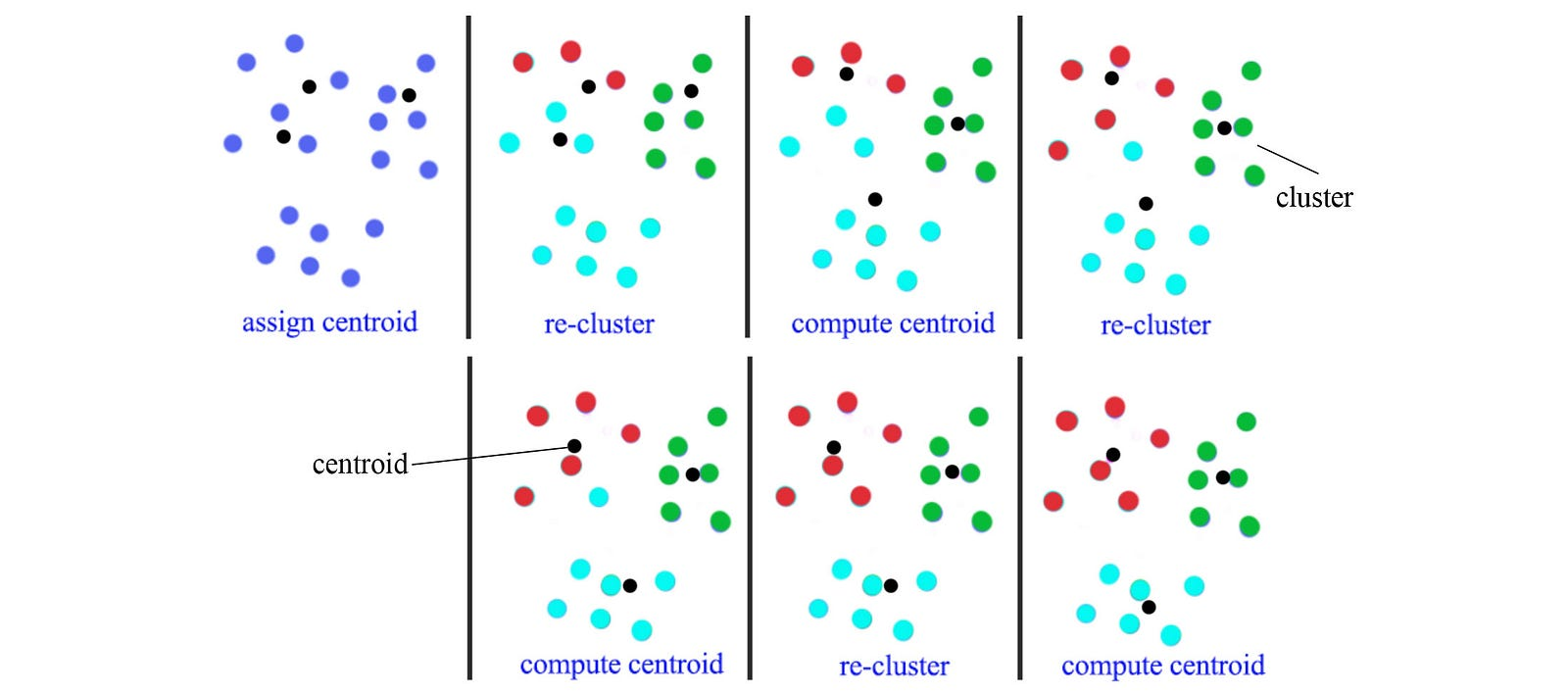k=3 (3 clusters)

We can repeat the process with different random seeds and use the total Euclidean distance in selecting the best model.

Convexity

The cost function for the K-mean clustering isSource

where cᵢ is the cluster assignment for the data point i and μⱼ is the centroid of the cluster j. K-mean clustering algorithm improves the clustering cᵢ and μⱼ in alternative steps. Therefore, the cost decreases monotonically. However, the cost function is non-convex. Therefore, the algorithm reaches a local optimal only. Different random seeds will likely result in different clusters. But with reasonable random initialization of the initial guess of the cluster’s centroids, it should produce a high-quality result.

Choosing K

So what is the proper value of K on the K-mean clustering? If we have N data points in the sample, we can train the model to have zero cost if we use N clusters. But this is not we want. So the first choice of K may determine by an explicit goal. For example, we want to manufacture a t-shirt with size XS, S, M, L, and XL. So K will be 5. Alternatively, we can study how fast the cost is dropping. We can stop at the elbow point knowing that further drops in cost will have a far less return.

We can also monitor the gap in error between the training dataset and the validation dataset. If the error in the validation training dataset is flattened or increased, we should not increase K further.

K-median clustering

Use median instead of mean for clustering to avoid outliners. We use L1 in measuring distance and cost.

K-means++ clustering

In K-means clustering, instead of initializing K centroids randomly at the beginning, we randomly select one centroid at a time. For the next centroid, we still select it randomly but with a higher preference for data points further away from the previous means. Here is the algorithm.

Bisecting K-means

We split each cluster into two but only commit the one that reduces the cost the most.

Gaussian mixture model (GMM)

A Gaussian mixture model is a probabilistic model that assumes all the data points are generated from a mixture of Gaussian distributions.

For K=2, we will have 2 Gaussian distributions G₁=(μ₁,σ₁²) and G₂=(μ₂, σ₂²). We start with random initialization of parameters μ and σ and compute the probability on which cluster that a data point may belong to. Then, we recompute the parameters μ and σ for each Gaussian distribution based on this probability. The data points are re-fitted to different clusters and the Gaussian parameters are re-calculated again. The iterations continue until the solution converges. Let’s detail this method using the EM algorithm.

Expectation–maximization (EM) algorithm (GMM)

The EM algorithm alternates between performing an expectation estimation (E-step) and a maximization (M-step). E-step computes

The probability p is computed by using the distance between xᵢ and μ of the corresponding cluster using Gaussian distribution.

We can compare q(aᵢ) and q(bᵢ) for xᵢ and choose which cluster that it should belong to. After all the computation, the training data is either assigned to a or b. Then we recompute μ and σ for each cluster according to the data points that it owns. However, this is not exactly done in GMM. Instead of assigning a data point to either one of the clusters, we keep the probability q(aᵢ) and q(bᵢ). We use that as a weight on how much influence xᵢ has in calculating μ and σ for the corresponding cluster. For example, the mean of cluster A is the weighted average of all data points with the weight equals q(aᵢ). A probabilistic model often has a smoother cost function with smaller curvature. This smoother surface stabilizes the training. By not assigning xᵢ deterministically to a cluster, we allow the training to converge faster. Here is the algorithm for GMM.

Here will have the proof of GMM using the equations from the EM algorithm. Below is the EM algorithm for any mixture models.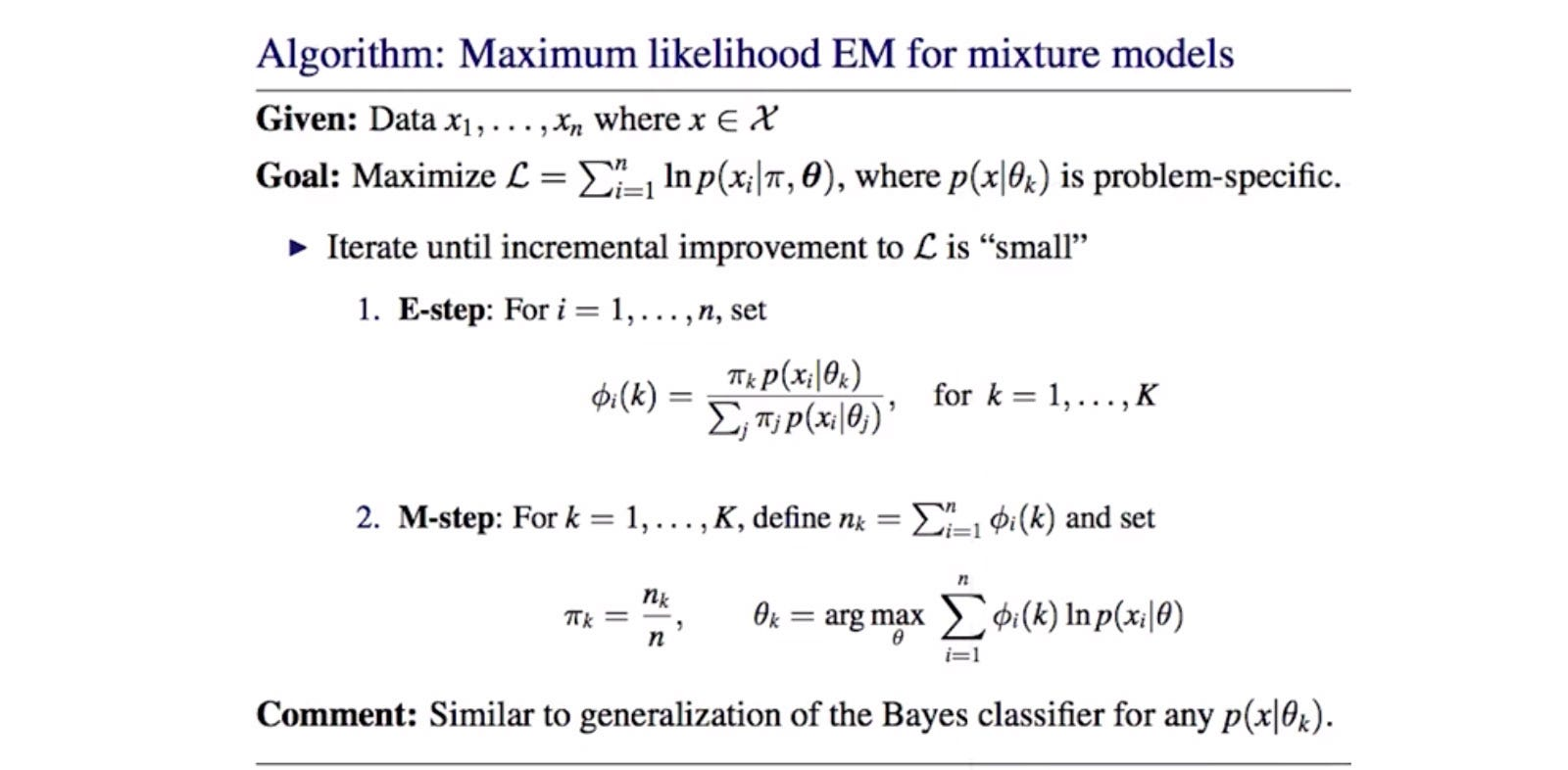Source

For GMM, the data distribution is assumed to be Gaussian distributed.

Self-organizing maps (SOM)

SOM produces a low-dimensional representation for high-density input. For example, we want to compute 39 index colors to represent the RGB values of an image. We start with 39 nodes with each node represented by a weight vector having the same dimension as the input (dim=3). So for each node, it is holding an RGB value.

Intuitively, we initialize all nodes randomly. Then we go through all data points in the training data set (every pixel of the image). We locate the node closet to the current data point RGB value. We update the RGB value of this current node and its neighbor towards this RGB value (with a less impact as we move away). As we go through the training data, the value of the nodes change and more representative to the color of the image pixels. Here is a recap.

• We random sample a data point (a pixel) from the image.
• We locate a node with weight as close as the input using L2 distance.
• We update the weight for surrounding nodes to match closer to the input. But the changes drops as we move away from the center.
• We sample the next data point and repeat the iteration.
• Eventually, the weight in each node represents the RGB value of the index color.

We use a learning rate to control the change and the change will decrease over distance and also decreases over time.

Density-based clustering (DBSCAN)

K-means clustering cannot discover manifold. Density-based clustering connects neighboring high-density points together to form a cluster. Intuitively, we gradually add neighboring points to a cluster. Such an approach allows the structure to grow slowly in discovering the neighbors as well as the manifold.

A data point is a core point if there are m reachable points within the radius r. A cluster is formed by connecting core points (the darker green) that are reachable from each other core points.

The green cluster is formed by the following method:

If we have a lot of data points, compute the distances for one data point to another is expensive. Instead, data points are partitioned into regions. We connect points that are in the same or adjacent grid regions only.

Density-Based Hierarchical Clustering

The hardest part of density-based clustering is determining the radius r.

Alternatively, we can use a top-down hierarchy approach in breaking a large cluster into sub-clusters. So we can start with a large r and gradually decrease it as we break down a cluster in the hierarchical structure.

Hierarchical clustering

There are many other Hierarchical clustering approaches, including top-down or bottom-up. These are the algorithms.

Ensemble Clustering (UBClustering)

Ensemble Clustering runs K-means m times with different random seeds to produce m models. If a simple majority of models agrees two points should belong to the same cluster, we make sure they are. Ensemble Clustering starts with no cluster,

• if two points should be together but do not have any cluster assignment, we create a new cluster for them.
• if one is assigned but not the other, we put the un-assigned to the cluster holding the assigned one.
• if they are assigned to different clusters, we merge both clusters together.

Canopy clustering

Clustering is expensive. But we can apply Canopy clustering to form some form of clusters before applying a more expensive method to refine it.

### Documents

TF-IDF

TF-IDF scores the relevancy of a document on a search query. To avoid exploitation of the search term, the score decrease if the terms commonly exist among documents. Therefore, it will score higher if the term is not common.

### Decision Tree

Technically, we are dissecting the input space binary at each decision stump.

A decision tree is also called Classification and Regression Trees (CART). A decision tree is easy to interpret, easy to prepare data and fast for inference. Since we use a greedy method in selecting decision stump and the branching conditions are simple, the model may not be too accurate, high variance and/or overfit easily.

For regression, we split the data at a specific feature with the value greater than a specific threshold. We try different features and threshold values combination by brute force and selects one that reduces the L2 error the most greedily. The prediction will be the mean or the median of the data points in the branching data set.

In a classification decision tree, we can use different criteria in choosing a decision stump. For example, we can compute the classification error, the Gini index or the entropy (details later). The tree prediction will be the majority vote by the branching data set.

Before choosing the decision stump, we can create a scatter plot matrix to spot the correlations between properties to give us some insights on how to split the tree.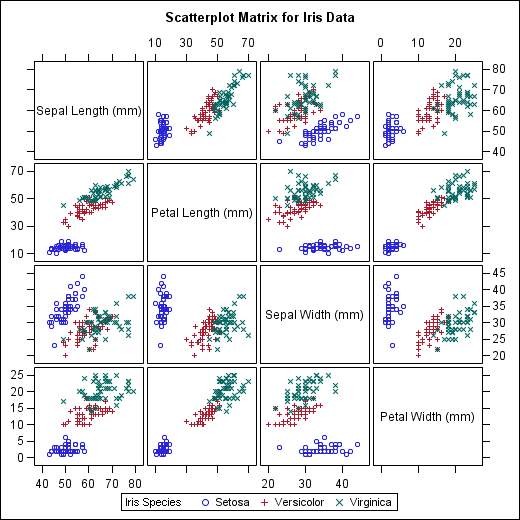Source

Next, we will discuss the details in selecting the decision stump.

Gini index

If we know 90% of the data belong to class i and the remaining 10% belongs to class j, we can make random guesses on the labels using the same ratio. In fact, we can do reasonably well (82% correct). Gini index measures how likely we are wrong in making predictions with such a scheme. If the data is very predictable, the Gini index will be low.

Example,

If the data from each branch belongs to the same class, Gini index equals zero. To pick the decision stump, we choose one with the lowest weighted Gini index.

Information Gain

We can pick the decision stump with the highest information gain.

In other words, we want the smallest conditional entropy after the branching.

Intuitively, after the branch we want the entropy to be the smallest and therefore it is the most predictable.

For example, we want to predict whether we want to play tennis or not. Below, it is the calculation on the information gain and what is the information gain if we choose windy (true or false) as the decision stump.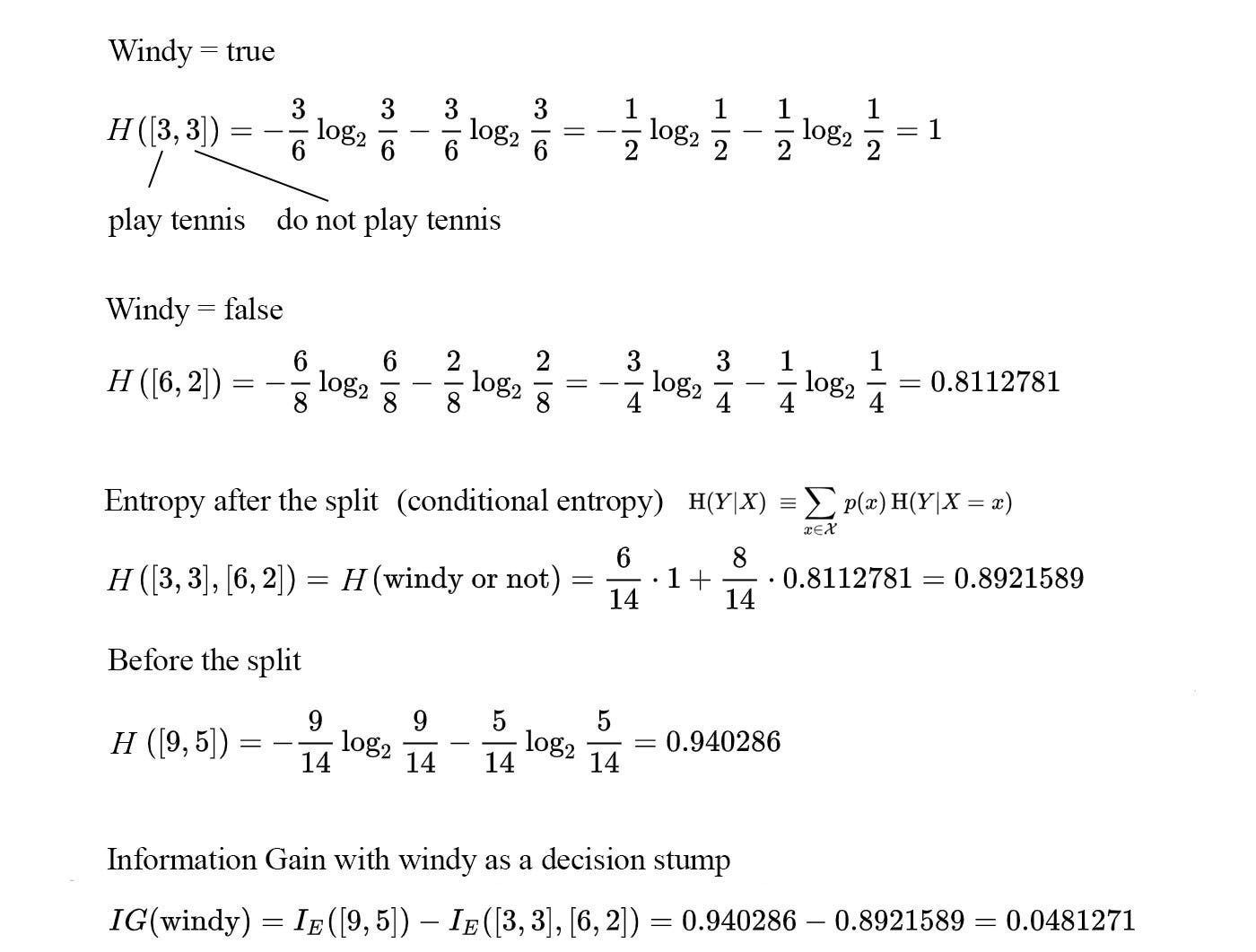Example modified from source

Then, we move on to other possible stump and select greedily for the one with the highest value.

Variance reduction

Variance reduction works with continuous space. It measures the variance before and after the branch. We want to choose a decision stump with the largest variance reduction.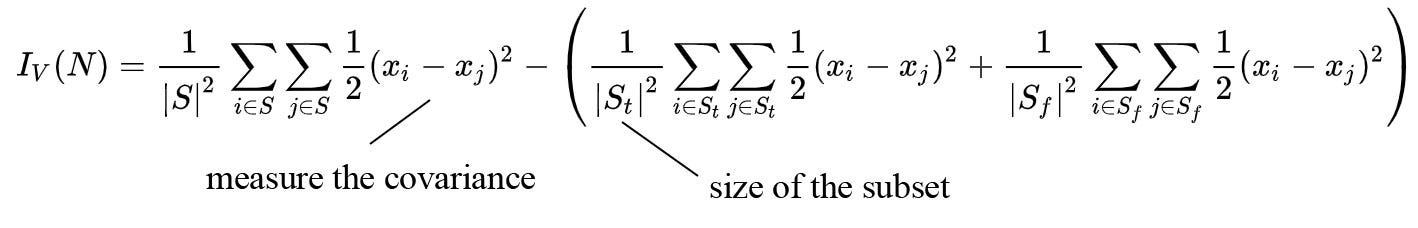Modified from source

Pruning

The following data points will take at least 4 levels of decision tree before the leaf node contains all red or blue dots. Therefore, if we use the progress to determine whether we should stop the splitting, we would stop prematurely. In this example, we will see no progress until we reach the fourth level.

For the decision tree, we can purposely grow the tree deeper and use pruning to trim the tree backward instead.

Overfitting

For the classification problem, we grow the tree until the data points for each branch belong to the same class or have the same input attributes. Due to noise in the data, this approach overfits the model easily. One possible solution is limiting the depth of the tree. Alternatively, as we mentioned before, we can prune the tree after it is built. We can start from the leaf and goes upward. We will remove the decision stump if the validation performance for the testing data improves.

Other approaches to limit the depth of the tree include

• Have a hard upper limit on the tree depth.
• Stop further branching if the number of data points drops below a threshold.
• Require each leaf node to have a minimum number of data points.
• Set a maximum number of the leaf node.

Chi-square test

We can also verify whether the decision stump is statistically significant with the Chi-square test. Chi-square measures the difference of data distribution between its parent and its children and evaluates whether the difference is mainly by chance within a certain confidence level (say 95%). If we think the difference in the distribution is easily explained by just simple chance, we may decide to take the stump out.

Example,

If Chi-square is smaller than a threshold T, we remove the decision stump because the difference in the data distribution is not statistically significant. (We often consult a table or a Chi-Square distribution calculator in finding the value T for the corresponding confidence level.)

We can use the Chi-square value in deciding the decision stump. We will pick the one with the highest value.

Weak learners

In ML, we can build a weak learner (models with less complexity) to avoid overfitting. For decision trees, we can restrict the depth of the tree and the number of input features to lower the model complexity. However, these learners are likely biased. As the Art of War said if you have the right commands and disciplines, you can turn 180 concubines into military companies. (A sharp contrast to the belief in the high-tech industry that you have to hire the best-of-the-best.) In ML, we can bundle models together in making predictions. If they are trained independently such that their correlation is small, they are unlikely making the same mistakes. A simple majority vote can cancel errors and create strong predictions. Next, we will talk about methods in “bundling” weak learners.

Ensemble methods

There are many combinations in ensembling models, including different algorithms, different seeds and configurations, and different models saved at different iterations. If cares are taken in avoiding strong correlations, we can use majority vote, average or weighted average, or median in making predictions.

Stacking

In the first round, we use multiple ML methods to make predictions. Then we feed these predictions as input to another model for the final decision.

Bagging (Bootstrap aggregated)

Bootstrapping is a general technique on random sampling with replacement. We select data from a data set. But the picked data can be reselected again (replacement). i.e., we select our data from the same set of data again and again.

Bagging (Bootstrap aggregated) simply applies this technique in collecting data points with random sampling and replacement. The data points sampled can be repeated or missing in this round of the training dataset. This reduces the correlations among trained models and avoids making the same mistakes. If we also lower the depth of the tree, we can also avoid overfitting. Then we can aggregate the results from all the models.

For example, we can have B bootstrap datasets each containing n data points. Each bootstrap samples with replacement from the original dataset of size n. We can compute the median of each bootstrap and then we estimate the median by computing a mean from all the bootstrap datasets. The mean and the variance of the estimation is:Source

Random forest

As the number of the bootstrap datasets increases, the performance of the ensemble trees plateau because many of the datasets are highly correlated. Random forest uses bagging mentioned above. In addition, each model is only trained with a randomly selected subset of features. Usually, only the square root number of features are selected in building each model. This further reduces the correlation of models. Different models will find different ways of determining a class. Combining it with bagging, it improves the accuracy of the ensemble methods.

Boosting

We should always learn from our mistakes. When we build our first model, we sample data from the training set uniformly. But for the next iteration, we sample data points that have the wrong predictions more frequently. With a better focus on where we are lacking, the second model should fare better in those areas. After k iterations, k models are built. And in each iteration, we keep track of the accuracy of the model and use this to compute a weight. During inference, we use these weights to compute a weighted average for the prediction using these models.

AdaBoost

Here is the AdaBoost which uses the boosting technique.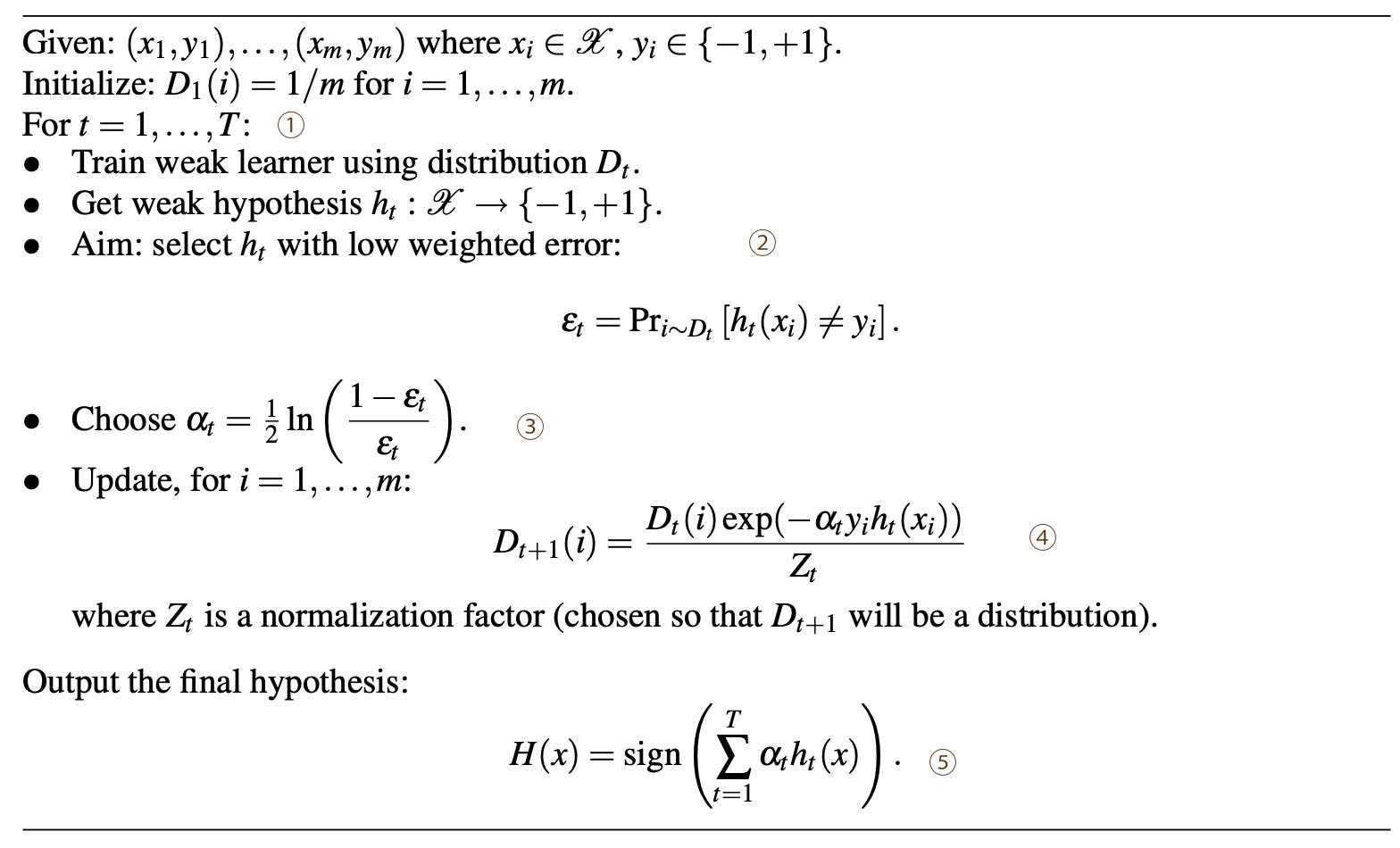Source

Here is the flow of the algorithm.

① Build one model in each time iteration.

② Select a model with the lowest error.

③ Compute α corresponding to the accuracy of this model.

④ We sample data with more emphasis on those that have the wrong prediction.

⑤ After the training, we use all T models to make independent predictions. The aggregated result will be a weighted average according to the accuracy of each model.

In general, we sample the misclassified data with a larger sampling weight for the next iteration.

The upper bound of the training error for the AdaBoost is (proof)Source

Even though we may learn a weak learner in each step, the error decreases significantly if we have learned enough models.

Gradient-Based Model

In AdaBoost, we learn from data points that fare badly. In Gradient-based model, we model our errors in our predictions. Let say we have a training data set y = [ f(100), f(200), …, f(800)] for input 100, … to 800. Now, we want to build a model for f. First, we model f with the average of y. This is a good start. Next, we compute the residual (error) in our prediction and model it.

We continue to build simple models out of the residuals and our prediction will simply add all the model results together.

Let’s do it graphically.

Here is one possible way of building a regression tree.

But there are other possibilities. Instead of building a model for the residual, we build a model for the sign of the residual only.

If the learning rate is low, the second solution requires us to build more models. But gradually, the results of these models will add up to make good predictions. What is the difference between these solutions and why do we call it gradient-based? If we compare these gradient-based models with gradient descent, they look similar except with a different sign.

As shown below, if we take the derivative of different loss functions, the gradient of the cost function is just the negative of the term that we model the residual in our examples. Updating F based on the models above corresponds to updating F based on the gradient of the chosen cost function.

So we can broaden the concept of the gradient-based method into other cost functions, like Huber loss. The general algorithm will be: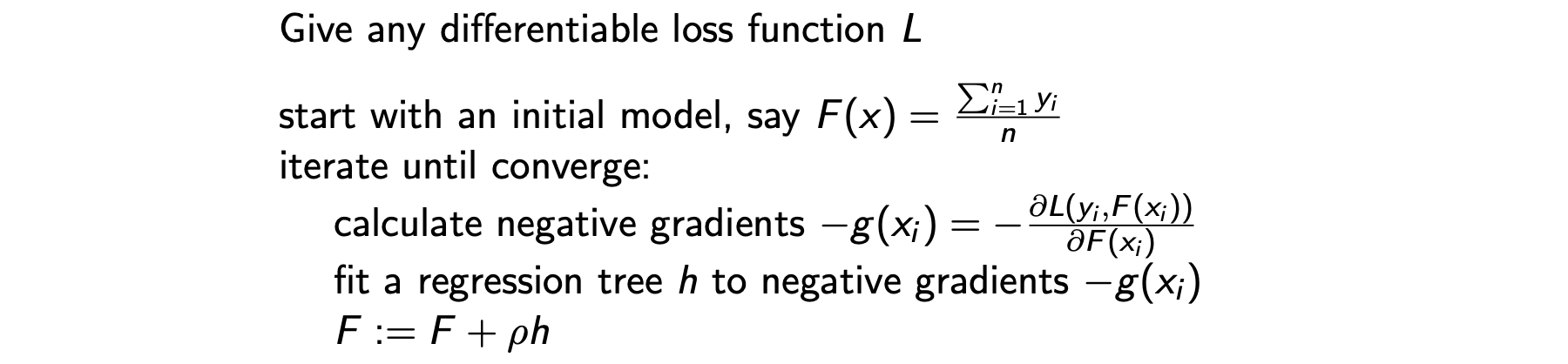Source

Local beam search

The searching space, like the GO game, is huge. In the Local beam search, we only explore the top most promising searches.

Regression tree

Besides classification, we can use a decision tree to break up the model into sub-cases and solve each leave node with a regression model.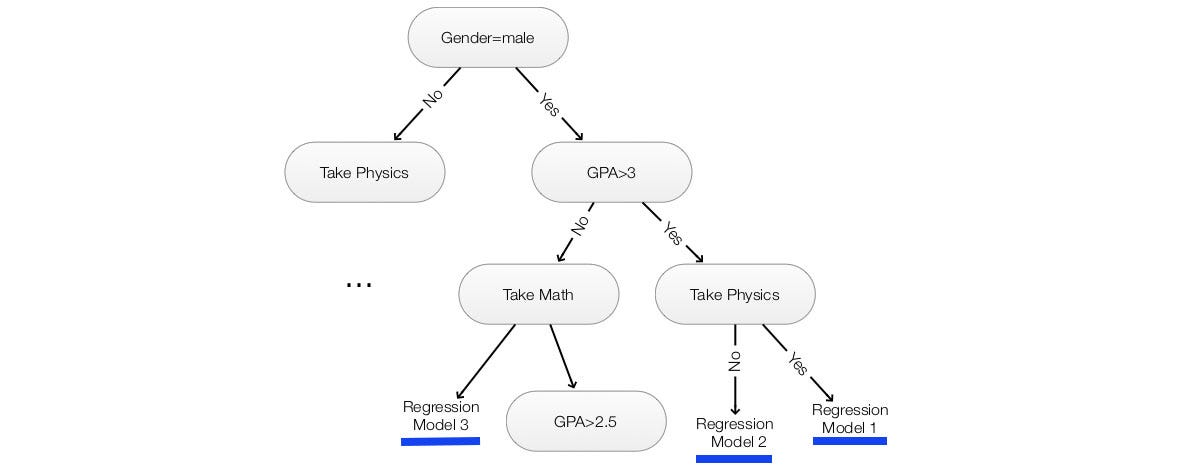Use a linear regression model to make predictions inside a decision tree

### Example of supervised & unsupervised learning

Supervised learning builds a model f in predicting a label given an input (y=f(x)) with a training dataset containing pairs of the input and the corresponding label (xᵢ, yᵢ). Conceptually, supervised learning is the study of P(y|x). Unsupervised learning finds patterns and trends of the training data. No label is attached. We can generalize it as the study of P(x). In K-means clustering, we approximate P(x) with the K centroids. Unsupervised learning includes clustering, matrix factorization, and sequential models.

In HMM, we are interested in the internal states. For example, in speech recognition, our observation is the audio and the internal state is the corresponding words. Since we do not capture the audio into words in HMM, it can be classified as unsupervised learning. However, the line drawn between supervised learning and unsupervised learning can be vague and sometimes not very important.

### Model complexity

We risk overfitting when we increase the model complexity, for example, the number of Gaussian models in GMM, the number of hidden states in HMM, or the rank in the matrix factorization, etc … As the model complexity increases, the training error diminishes. However, when we validate the model with a validation dataset, we will realize the validation error will increase instead. In DL, we often dedicate a portion of data for validation purpose only. In ML, cross-validation or bootstrapping data (random sampling with replacement) is common in selecting training data. We use the dedicated validation dataset, hold-out data set or the full dataset respectively in choosing the model complexity. Similar to the regularization, we can add a penalty to the cost function.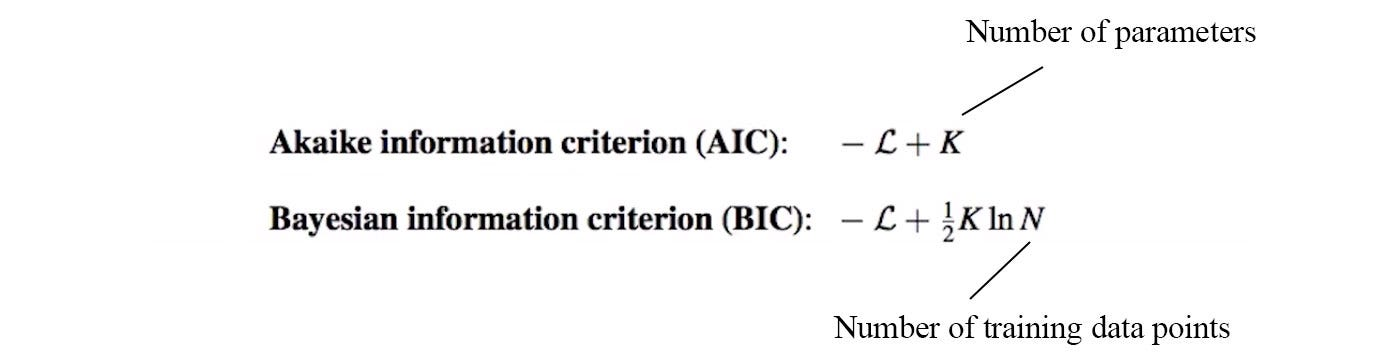Modified from source

Therefore, we only increase the model complexity only if the cost return is justified. Here is the proof in justifying BIC.

### Energy-based model

Some research papers explain their concepts through the energy-based model. So it may be nice to have some understanding of it.

With an energy function E(x), the probability distribution for the configuration (state) x is defined as

For such a model, high energy E(x) leads to a low possibility for x. We want to build an energy function that can maximize the P(x) of the training dataset. A partition includes all possible non-overlapping events. Therefore, we call Z above the partition function. Basically, it normalizes the probability between 0 an 1. It is not easy to compute Z for a system with many states. Sampling is often applied to solve those problems. Next, we will focus on defining the energy function.

Boltzmann Machine

To increase the expressiveness of an energy model, we add non-observed variables (hidden units) into a Boltzmann Machine. This model composes of visible units (white) and hidden units (blue) that are fully connected. Each unit is in one of the binary state sᵢ∈{0,1} with W modeling the undirected connection between them. The energy function E(X) and probability distribution P(X) for the Bolzman Machine is defined as

If sᵢ and sⱼ are positively related, we want Wⱼ>0. If they are negatively related, we want Wⱼ<0. Conceptually, we want to turn on/off a unit according to the other units and their correlations. In a Boltzmann Machine, it extracts the latent factor of the inputs with the largest coherence with the training dataset. However, each node is interconnected with each and is very hard to train.

Restricted Boltzmann Machine (RBM)

RBM extracts latent feature h from the input v only. It is more restrictive but the model is much simpler than a Boltzmann machine because visible units are not connected with each other, the same for the hidden units. The energy E(v, h) for the configuration (v, h) equals

To train the model, we optimize the maximum log-likelihood of the training data. Here is the gradient for updating w.

Part of the logic in deriving the gradient can be found here.

Contrastive Divergence

However, finding the expectation for the second term is not easy. One possibility is to estimate it with alternating Gibbs sampling.

Starting with any value for v, as we continue to iterate, v and h will resemble the P(v, h) of the model. However, this is time-consuming. An alternative contrastive divergence method is proposed with

and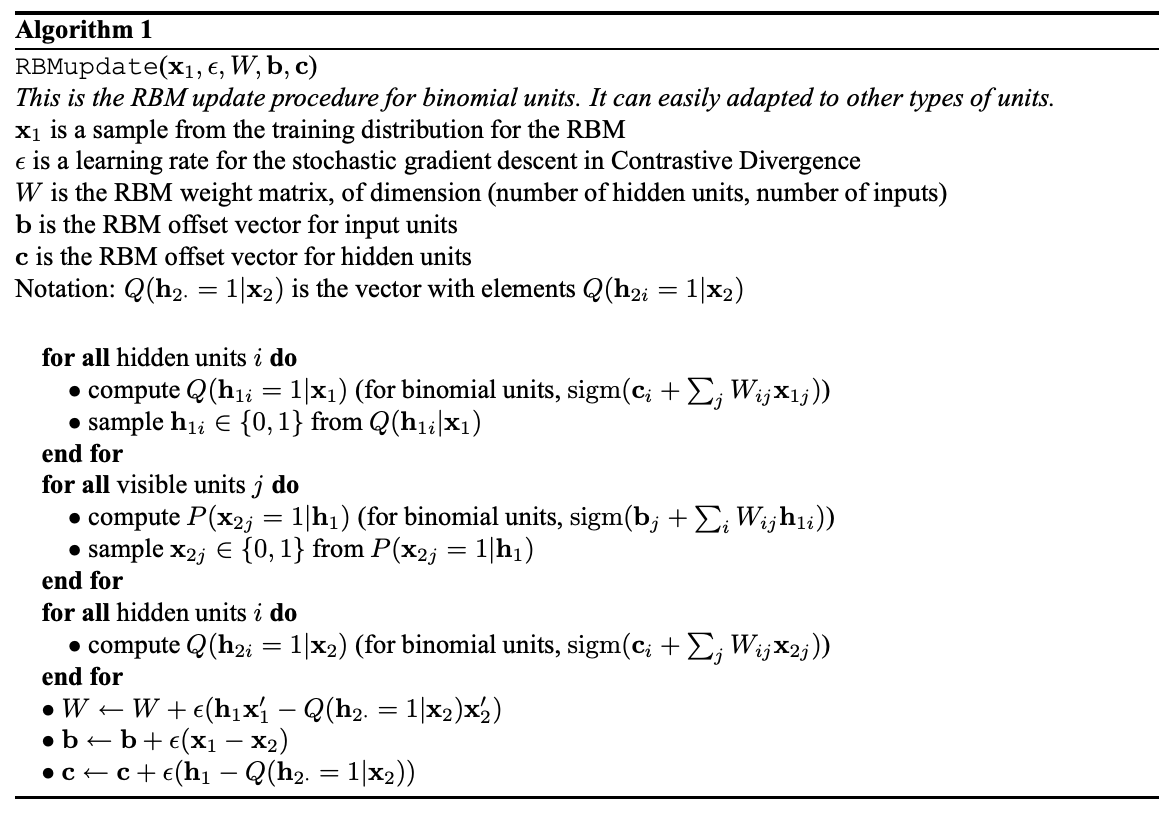Source (x’ is column form of x)

More details for the gradient used in the contrastive divergence can be found here. The equation is slightly different from the one above. To understand different variants of the algorithm. Please refer to reference 1 and reference 2 for more information.

### CNN

We use k × k convolution in each layer to extract feature maps. With stride or max-pooling, we reduce the spatial dimension gradually. (Since there are many good resources on CNN, the description here is extremely brief.)

### LSTM & GRU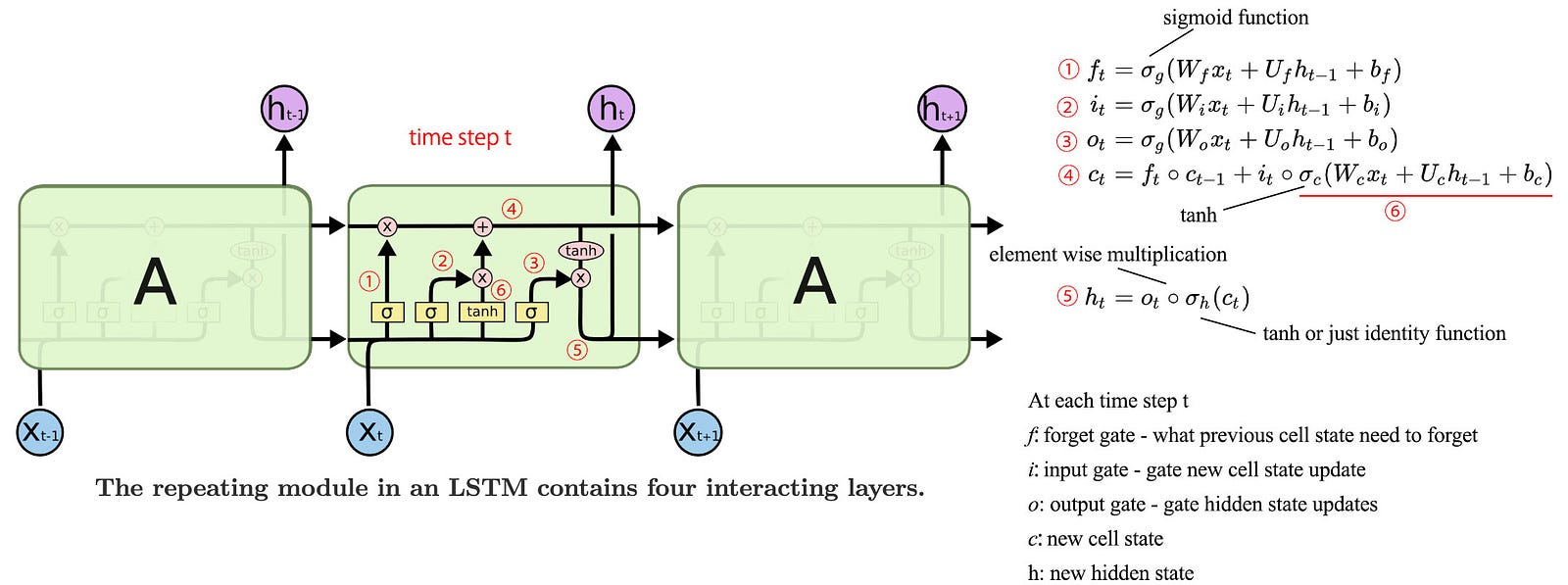Modified from source 1 & 2

In LSTM, we have three gates (forget, input & output gate — with symbol ⊗
above) in gating information. It has the form of

which applies a sigmoid function to the current data input and the previous hidden state after multiplying with different W values. To create a new cell state, we forget part of the old cell state and pass new cell information through the input gate.

We then apply the output gate to the new cell state to create the new hidden state.

Compare with LSTM, GRU does not maintain a cell state C and use 2 gates instead of 3.

### Process

Below is the process flow for an ML/DL project.

Clip art source